## Access Answers to NCERT Class 7 Maths Chapter 14 – Symmetry Exercise 14.2

### Access Answers to NCERT Class 7 Maths Chapter 14 – Symmetry Exercise 14.2

1. Which of the following figures have rational symmetry of order more than 1: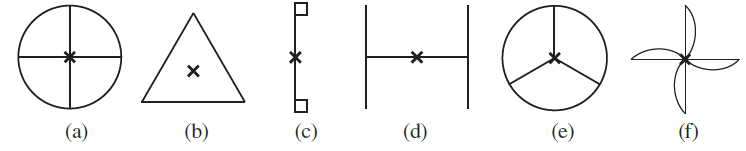Solution:-

(a)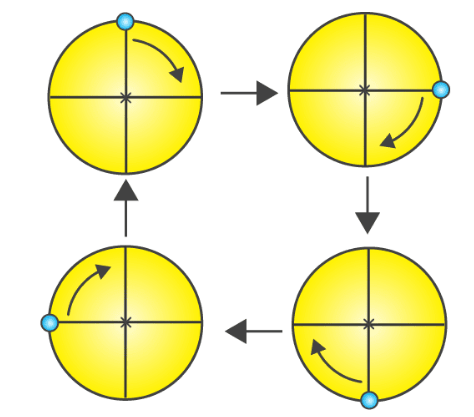So, the above figure has its rotational symmetry as 4.

(b)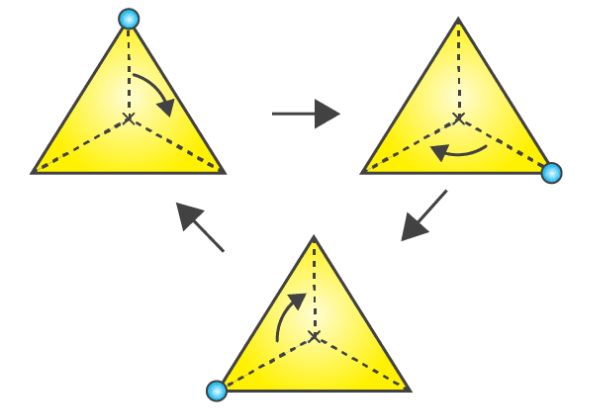So, the above figure has its rotational symmetry as 3.

(c) So, the given figure has only one rotational symmetry.

(d)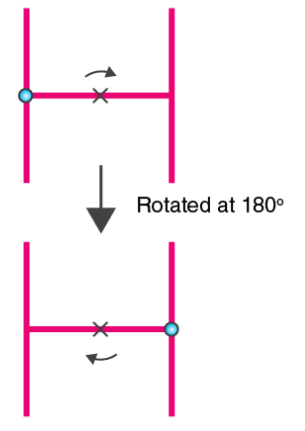So, the above figure has its rotational symmetry as 2.

(e)So, the above figure has its rotational symmetry as 3.

(f)So, the above figure has its rotational symmetry as 4.

By observing all the figures (a), (b), (c), (d), (e) and (f) have rotational symmetry of order more than 1.

2. Give the order of rotational symmetry for each figure:

(a)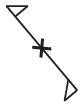Solution:-The above figure has its rotational symmetry as 2.

(b)Solution:-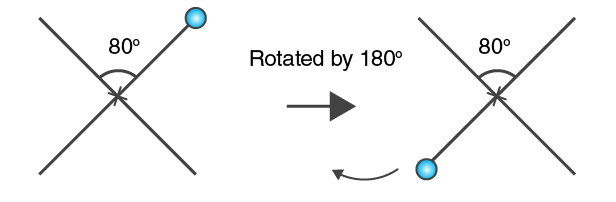The above figure has its rotational symmetry as 2.

(c)Solution:-

98″ alt=”NCERT Solutions for Class 7 Maths Chapter 14 Symmetry Image 98″ />

The above figure has its rotational symmetry as 3.

(d)Solution:-The above figure has its rotational symmetry as 4.

(e)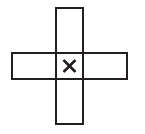Solution:-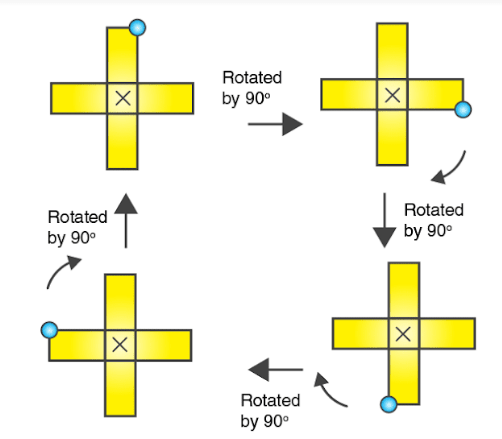The above figure has its rotational symmetry as 4.

(f)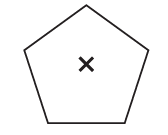Solution:-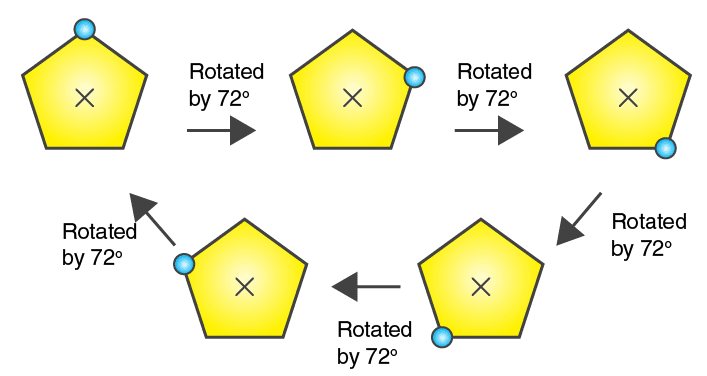The above figure has its rotational symmetry as 5.

(g)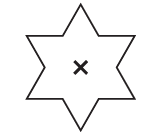Solution:-The above figure has its rotational symmetry as 6.

(h)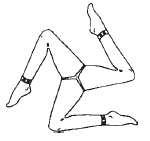Solution:-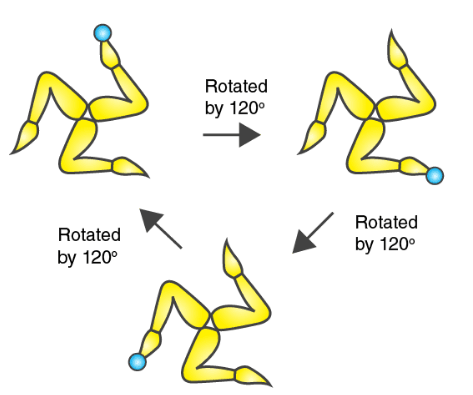The above figure has its rotational symmetry as 3.